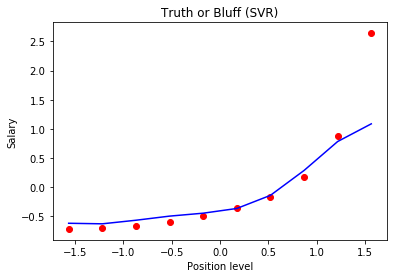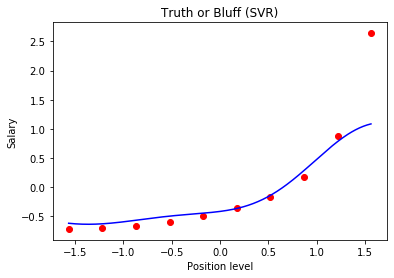0%

We are going to implement a linear support vector regression

Support vector regression is a type of support vector machine

SVR is tube like structure. We do not care about the points in the tube whereas we care about the points outside the tube as it determines the tube position.

Here is how a SVR looks like:For this model we will be using decision tree to predict a salary of an employee with position level of 6.5

Note: We also use a non linear SVR that looks like this:For this example we will use a RBF kernel support vector machine. We use kernel to determine the linearity of out support vector machines. The kernel we mostly use in the non linear support vecotor is RBF kernel.

## Let us start

In :
import numpy as np
import pandas as pd
from matplotlib import pyplot as plt


## Importing the dataset / Create Own

In :
df = pd.DataFrame({'Position': ['Business Analyst', 'Junior Consultant', 'Senior Consultant', 'Manager', 'Country Manager', 'Region Manager', 'Partner', 'Senior Partner', 'c-level', 'CEO'], 'Level':[1,2,3,4,5,6,7,8,9,10], 'Salary': [45000, 50000, 60000, 80000, 110000, 150000, 200000, 300000, 500000, 1000000]})
x = df.iloc[: , 1:2].values
y = df.iloc[:, 2].values
y = y.reshape(-1,1)
print(df)

            Position  Level   Salary
1  Junior Consultant      2    50000
2  Senior Consultant      3    60000
3            Manager      4    80000
4    Country Manager      5   110000
5     Region Manager      6   150000
6            Partner      7   200000
7     Senior Partner      8   300000
8            c-level      9   500000
9                CEO     10  1000000

In :
print(x.T, '\n',y.T)

[[ 1  2  3  4  5  6  7  8  9 10]]
[[  45000   50000   60000   80000  110000  150000  200000  300000  500000
1000000]]


## Feature Scaling

In [ ]:
from sklearn.preprocessing import StandardScaler
sc_x = StandardScaler()
sc_y = StandardScaler()
x = sc_x.fit_transform(x)
y = sc_y.fit_transform(y)


## Fitting Linear Regression to the dataset

In :
from sklearn.linear_model import LinearRegression
lin_reg = LinearRegression()
lin_reg.fit(x, y)

Out:
LinearRegression(copy_X=True, fit_intercept=True, n_jobs=None,
normalize=False)

## Fitting SVR to the dataset

In [ ]:
from sklearn.svm import SVR
regressor = SVR(kernel = 'rbf')
regressor.fit(x, y)


## Predicting a new result

In :
y_pred = regressor.predict([[6.5]])
y_pred = sc_y.inverse_transform(y_pred)


## Visualising the SVR results

In :
plt.scatter(x, y, color = 'red')
plt.plot(x, regressor.predict(x), color = 'blue')
plt.title('Truth or Bluff (SVR)')
plt.xlabel('Position level')
plt.ylabel('Salary')
plt.show()## Visualising the SVR results (for higher resolution and smoother curve)

In :
X_grid = np.arange(min(x), max(x), 0.01) # choice of 0.01 instead of 0.1 step because the data is feature scaled
X_grid = X_grid.reshape((len(X_grid), 1))
plt.scatter(x, y, color = 'red')
plt.plot(X_grid, regressor.predict(X_grid), color = 'blue')
plt.title('Truth or Bluff (SVR)')
plt.xlabel('Position level')
plt.ylabel('Salary')
plt.show()In [ ]: